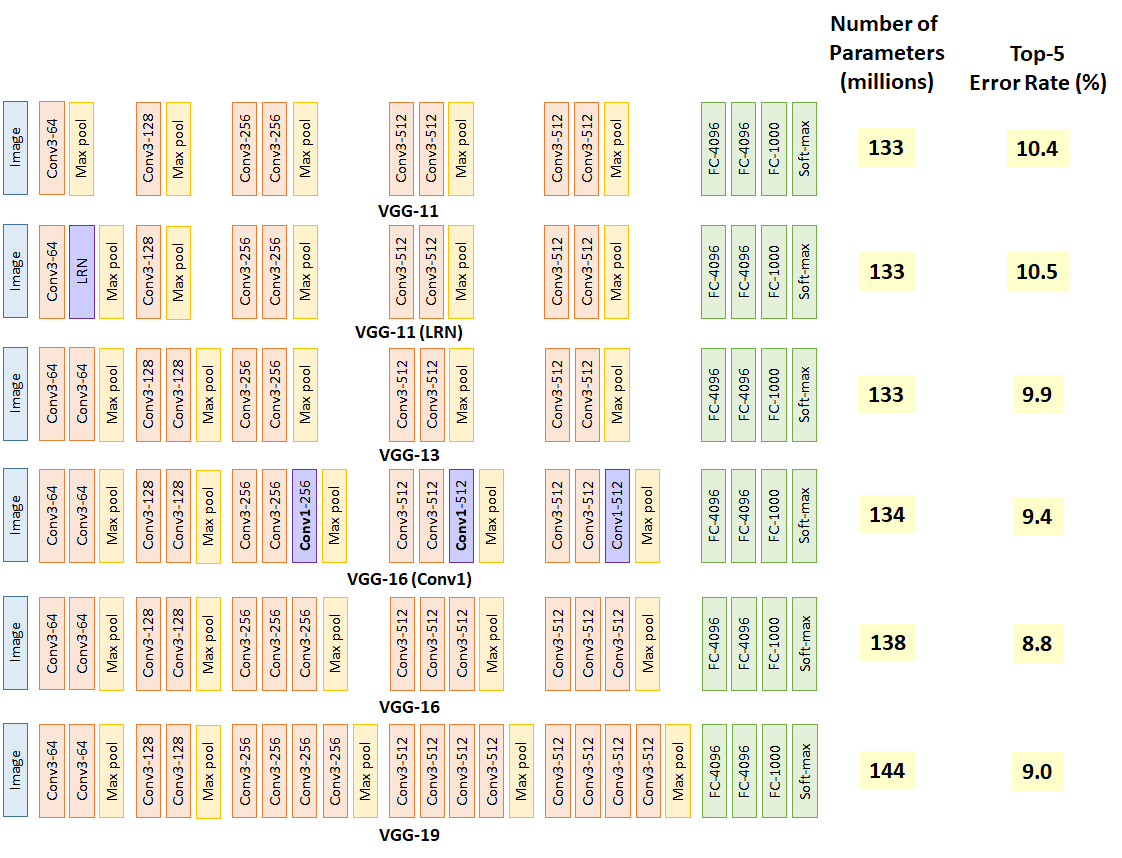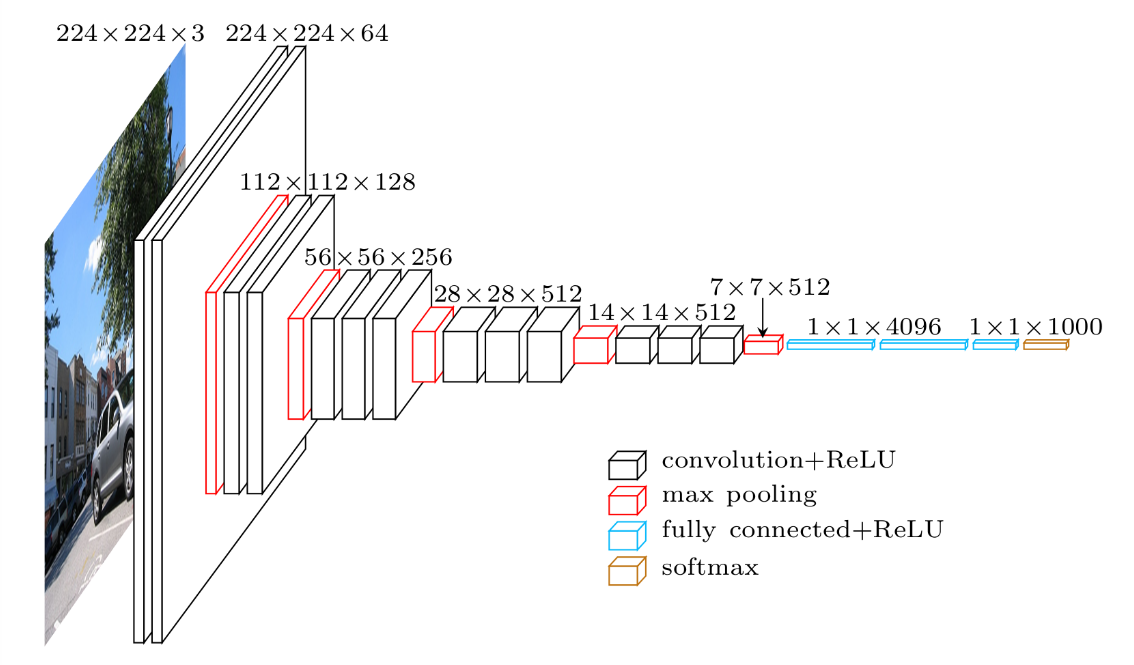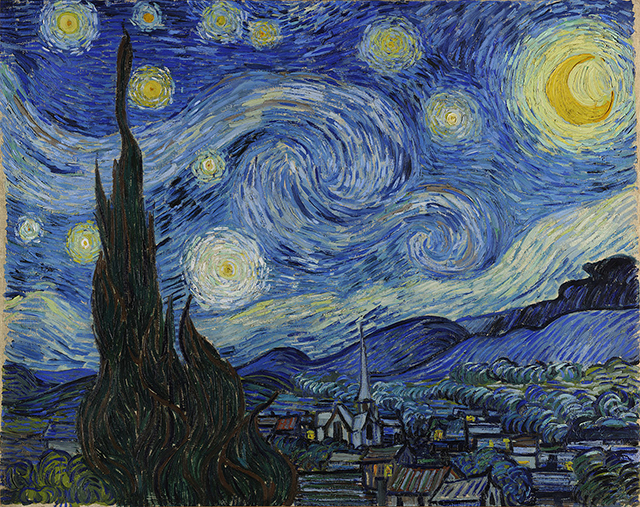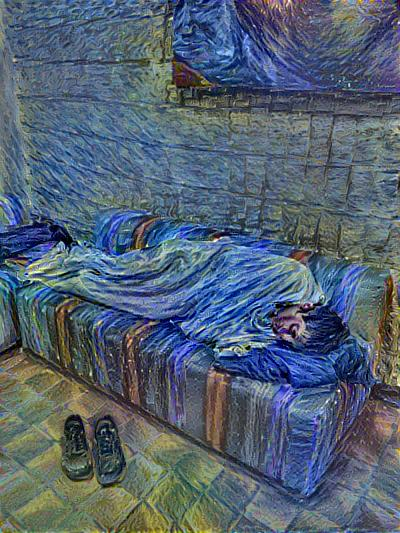# 从锅炉工到AI专家(8)

TensorFlow实务

#### ImageNet

ImageNet还连续举办了多届机器识别大赛，比赛结果一次次刷新机器学习识别纪录，最新的成绩已经超过人眼在图像识别方面的平均水平。诞生了很多优秀的算法或者模型。

• 大规模并准确标注的数据集用于训练、验证、测试
• 强悍的硬件，特别是有强劲的GPU帮助计算
• 更深的网络、更巧妙的网络组合及Dropout/ReLU算法的组合应用

#### vgg-19

VGG-19是2014年在ImageNet大赛中夺冠的算法，整体模型定义超过了19层的卷积及神经网络。下图是vgg系列网络的结构示意图：``````pip2 install scipy pillow
``````

#### 先看源码

vgg.py:

``````#这个程序相当于一个库，不会直接执行，
#所以开始没有用于脚本模式的标志
# -*- coding=UTF-8 -*-

import tensorflow as tf
import numpy as np
import scipy.io as sio

netmat_path = 'data/imagenet-vgg-verydeep-19.mat'

##定义卷积层
def _conv_layer(input, weight, bias):
conv = tf.nn.conv2d(input, tf.constant(weight), strides=(1, 1, 1, 1), padding='SAME')

##定义池化层
def _pool_layer(input):
return tf.nn.max_pool(input, ksize=(1, 2, 2, 1), strides=(1, 2, 2, 1), padding='SAME')

##定义全链接层
def _fc_layer(input, weights, bias):
shape = input.get_shape().as_list()
dim = 1
for d in shape[1:]:
dim *= d
x = tf.reshape(input, [-1, dim])
fc = tf.nn.bias_add(tf.matmul(x, weights), bias)
return fc

##定义softmax分类输出层
def _softmax_preds(input):
preds = tf.nn.softmax(input, name='prediction')
return preds

##图片处里前减去均值（归一化的部分工作）
def _preprocess(image, mean_pixel):
return image - mean_pixel

##加均值，用于还原可显示图片
def _unprocess(image, mean_pixel):
return image + mean_pixel

##构建cnn前向传播网络
def net(data, input_image):
#根据vgg19的标准定义深层卷积网络
layers = (
'conv1_1', 'relu1_1', 'conv1_2', 'relu1_2', 'pool1',

'conv2_1', 'relu2_1', 'conv2_2', 'relu2_2', 'pool2',

'conv3_1', 'relu3_1', 'conv3_2', 'relu3_2',
'conv3_3', 'relu3_3', 'conv3_4', 'relu3_4', 'pool3',

'conv4_1', 'relu4_1', 'conv4_2', 'relu4_2',
'conv4_3', 'relu4_3', 'conv4_4', 'relu4_4', 'pool4',

'conv5_1', 'relu5_1', 'conv5_2', 'relu5_2',
'conv5_3', 'relu5_3', 'conv5_4', 'relu5_4', 'pool5',

'fc6', 'relu6',
'fc7', 'relu7',
'fc8', 'softmax'
)

weights = data['layers']
net = {}
current = input_image
#枚举所有的层
for i, name in enumerate(layers):
#取名字的前4个字母作为本层的类型
kind = name[:4]
#根据conv/relu/pool/soft这几种情况，调用对应函数定义相应层
#每次定义层以上一层的输出为输入，将整个网络连接起来
if kind == 'conv':
kernels, bias = weights[i]
kernels = np.transpose(kernels, (1, 0, 2, 3))
bias = bias.reshape(-1)
current = _conv_layer(current, kernels, bias)
elif kind == 'relu':
current = tf.nn.relu(current)
elif kind == 'pool':
current = _pool_layer(current)
elif kind == 'soft':
current = _softmax_preds(current)

#处理最后的全连接层
kind2 = name[:2]
if kind2 == 'fc':
kernels1, bias1 = weights[i]

kernels1 = kernels1.reshape(-1, kernels1.shape[-1])
bias1 = bias1.reshape(-1)
current = _fc_layer(current, kernels1, bias1)

net[name] = current
assert len(net) == len(layers)
return net, layers

def predict(image):
global data,mean_pixel
#统一减去均值
image_pre = _preprocess(image, mean_pixel)
#拉成数组
image_pre = np.expand_dims(image_pre, axis=0)

#转成浮点矩阵
image_preTensor = tf.to_float(tf.convert_to_tensor(image_pre))

#定义深度VGG19神经网络及载入图片数据用于预测
nets, layers = net(data, image_preTensor)

#取分类层数据
preds = nets['softmax']

#从分类转成整数的索引，
#实际上.eval才是真正开始tensorFlow计算，以前那么多工作都是在建模型
predsSortIndex = np.argsort(-preds.eval())

#返回预测数据
return predsSortIndex,preds

#载入训练好的矩阵文件，
mean = data['normalization']
#获取图片像素均值用于图片的归一化
mean_pixel = np.mean(mean, axis=(0, 1))
``````

picRegn.py:

``````#!/usr/bin/env python
# -*- coding=UTF-8 -*-

import vgg
import os,sys
import numpy as np
import scipy.misc
import tensorflow as tf
import argparse

FLAGS = None

##读取图片并把图片尺寸归一化
def _get_img(src, img_size=False):
img = scipy.misc.imread(src, mode='RGB')
if not (len(img.shape) == 3 and img.shape == 3):
img = np.dstack((img, img, img))
if img_size != False:
img = scipy.misc.imresize(img, img_size)
return img.astype(np.float32)

##获取路径中文件列表
def list_files(in_path):
files = []
for (dirpath, dirnames, filenames) in os.walk(in_path):
files.extend(filenames)
break
return files

##获取文件路径列表dir+filename
def _get_files(img_dir):
files = list_files(img_dir)
return [os.path.join(img_dir, x) for x in files]

##获得图片的分类标签值
def _get_allClassificationName(file_path):
f = open(file_path, 'r')
f.close()
return lines

def main(_):
##加载ImageNet mat标签
lines = _get_allClassificationName('data/synset_words.txt')

images = _get_files(FLAGS.image_dir)  ##获取图片路径中文件列表
with tf.Session() as sess:
for i, imgPath in enumerate(images):
##加载图片并压缩到标准格式=>224x224x3色
image = _get_img(imgPath, (224, 224, 3))
predsSortIndex,preds = vgg.predict(image)

print('#####%s#######' % imgPath)
for i in range(3):   ##输出前3种分类
nIndex = predsSortIndex
classificationName = lines[nIndex[i]] ##分类名称
problity = preds[nIndex[i]]   ##某一类型概率

print('%d.ClassificationName=%s  Problity=%f' % ((i + 1), classificationName, problity.eval()))
sess.close()

if __name__ == '__main__':
parser = argparse.ArgumentParser()
help='Pic files folder path')
FLAGS, unparsed = parser.parse_known_args()
tf.app.run(main=main, argv=[sys.argv] + unparsed)
``````

#### 代码讲解``````\$ ./picRegn.py
#####images/leopard.jpeg#######
1.ClassificationName=n02128385 leopard, Panthera pardus
Problity=0.941652
2.ClassificationName=n02128925 jaguar, panther, Panthera onca, Felis onca
Problity=0.031439
3.ClassificationName=n02130308 cheetah, chetah, Acinonyx jubatus
Problity=0.024339
``````

vgg.py最主要的函数是net,其它函数都是围绕这个函数而工作的。在这个函数中，首先完整定义一个vgg19的模型。这个定义跟我们以前用的方式非常不同。在我们以前学习的时候，因为结构比较简单，都是直接逐行的用命令进行模型的搭建。而在这个19层的网络中，用手工搭建已经太繁琐了，因此用了一个字符串数组`layers`，将模型以字符串的形式存入数组。然后循环遍历这个数组，根据该层名称调用相应的子程序定义相应层的算法。并将每一层的输入，定义为上一层的输出，从而将网络逐层关联在一起，最终完成完整的网络。

vgg19的网络看上面展开的图反而不一定容易理解，我再转帖一张vgg-16网络的结构化示意图供参考（vgg-19网络除了层数其它基本类似）：#### 另一个脑洞

《A Neural Algorithm of Artistic Style》,这篇论文认为，既然深度学习网络识别图片的主要理论依据，是找出图片的各项特征。那这些特征，同艺术品的绘画风格是否有共通性呢？随后证明的确是可行的。在这里有了一个完整的实现，用于将一副风格鲜明的艺术品：vgg.py的代码对照我们上面的实现来读很容易理解，几乎都是相同的。所以这里补充一句，这一类的经典实现，保存到自己的代码库吧，就好像一把瑞士军刀，用的时候，拿出来简单改改就可以投产。
neural_style.py是主程序，主要定义和解析命令行参数，其中有大量的常量用于优化合成的效果。网上还有很多其它实现，但这个实现效果最好，跟这些复杂的参数有很大关系，因为显然作者使用这些配置灵活的参数已经进行了长时间的算法调优。主程序中其它的工作就是读写图片文件、基本的初始化性质的工作。
stylize.py是算法实现的主要部分，具体的实现如果有兴趣，建议先读一下论文，才能理解算法是如何实现的。

1. 使用特定公式将提取的风格矩阵的RGB格式转为灰度图；
2. 将灰度图再转为YUV格式；
3. 原图提取的特征矩阵从RGB转换为YUV；
4. 使用风格矩阵的Y部分，原图特征矩阵的U和V部分，重新组合为一张新图；
5. 将新图转回RGB,作为结果输出。补充一点，RGB分别代表红、绿、蓝应当大家都知道了，YUV分别代表Y(亮度)、及U/V两种(色度)信号。

(待续…)

#### 引文及参考